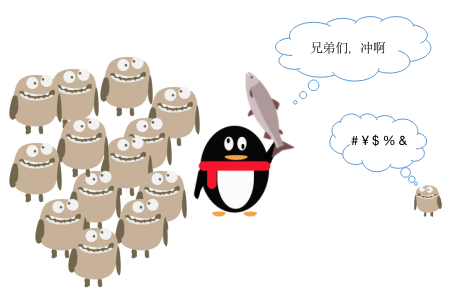BAT 面试之动态规划（二）腾讯笔试中的背包问题应用输入输出

// 一个例子
int n = 5;
int[] power = {4,1,1,1,5}; // 武力值数组
int[] cost = {1,2,2,2,3}; // 需要的金币数组
// 最少使用3枚金币，贿赂前两只怪物即可


分析

$P[i][j] = max(P[i-1][j],P[i-1][j-cost[i]]+power[i])$

$P[i][j]$ 使用 $j$ 枚金币闯过前 $i-1$ 只怪兽的关卡后携带的武力值。

优化与实现

$P[j] = max(P[j],P[j-cost[i]]+power[i])$

	/*
* 使用int数组表示中间结果
*/
private static int MinCoins(int n, int[] power, int[] cost) {
if (power.length == 0) return 0;
int max = 0;
for (int c : cost) max += c;  // 最多需要max枚金币

int[] P = new int[max + 1];
for (int p : P) p = -1;  // 初始化-1
for (int i = 0; i < n; i++) {
for (int j = max; j >= 0; j--) { // 从后往前更新
int temp1 = P[j] >= power[i] ? P[j] : -1; // 武力值要大于遇到的怪兽
int temp2 = (j >= cost[i]) && (P[j - cost[i]]!= -1) ? // 子问题必须已经解决
P[j - cost[i]] + power[i] : -1;

P[j] = temp1 > temp2 ? temp1 : temp2;
}
}
// 结果
int index = 0;
for (; index < max+1;index++) {
if(P[index] != -1) break;
}
return index;
}